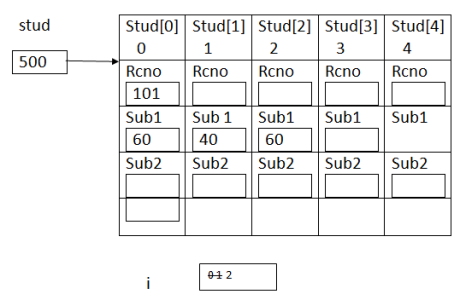Array of Objects in C++ Language
Ratings:
(4)
Views:191## Array of objects

Creating more than one object of similar type is called Array of objects in C++ creating an array of type class. syntax class-name array-name[size]

### // wap to find result 5 students

#include <iostream.h> class student { int no; int sub1,sub2; public read_student(); void find_student(); } void student read_student() { cout<<”Enter rno”; cin>>rno; cout<<”Enter marks of 2 subjects”; void  student :: find _student() { cout<<”\n<<rno<<”\t”<<sub|<<”\t”<<sub2; cout<<”\t Fail”; else cout<<”\t pass”; } void main() { student stud; int i; for(i=0;i<5;i++) stud[i].read_student(); for(i=0;i<5;i++) stud[i].find_result(); }   O/P :  input rollno 101 input 2 subject marks 604 input roll no 102 i/p 2 subject## Dynamic Array of object in C++

• Allocating memory for more than one object during runtime

Syntax <pointer-to-object> = new class-name[size];

• size can be constant or variable

### Example :// wap to read the details n persons and display

# include <iostream.h> class person { char name; char city; public : void read_person(); void print_person(); }; void person  :: read person() { cout<<”\n input name”; cin>>name; cout<<”\n input ciyt”; } void person :: print_person() { cout<<”\n”<<name<<”\t”<<city; } void main() { int n; person   *p; cout<<”\n input details of how many person”; cin>>n; p=new person[n]; int i; for(i=0;i<n;i++) { P[i].read_person(); for(i=0;i<n;i++) p[i].print_person(); delete P; } # include<iostream.h> { class player { char name; int runs; public : void read(); void print(); }; void player :: read() { cout<<”\n input name”; cin>>name; cout<<”\n input runs”; cin>>runs; } void player  :: print() { cout<< “\n”<<name<<”\t”<<runs; } void main() { int n; cout<<”\n input details of house many players”; cin>>n; player *p; p=new player[n]; int i; for(i=0;i<n;i++) p[i].read(); for(i=0;i<n;i++) (*(p+i).print()); delete P; P=NULL; }